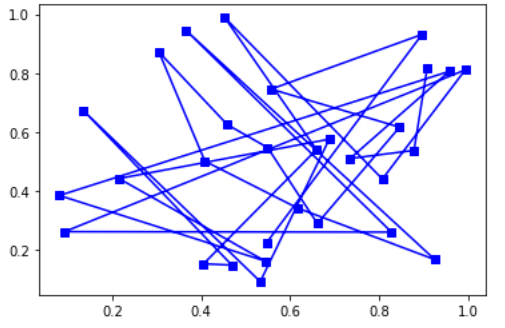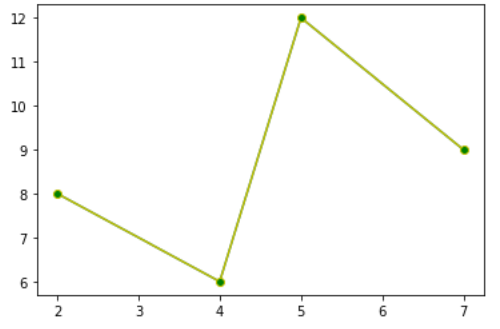Related Articles

# matplotlib.lines.VertexSelector class in Python

• Last Updated : 20 Aug, 2021

The matplotlib.lines.VertexSelector class belongs to the matplotlib.lines module. The matplotlib.lines module contains all the 2D line class that can be drawn with a variety of line styles, markers, and colors. matplotlib.lines.VertexSelector class is used to for managing the callbacks that maintain a list of selected vertices for matplotlib.lines.Line2D. The process_selected() function gets overridden by the derived classes for the picks.

Syntax: class matplotlib.lines.VertexSelector(line)
Parameter:

• lines: These represent the lines that are defined for the plotting.

Methods of the class:

1. onpick(self, event): The selected set of indices gets updated when the line is picked using this method.
2. process_selected(self, ind, xs, ys): By default it does the implementation of “do nothing” of the process_selected() method. The ‘ind’ represents the indices of the selected vertices whereas ‘xs’ and ‘ys’ are the coordinates of the selected vertices.

Example #1:

## Python3

 `import` `numpy as np``import` `matplotlib.pyplot as plt``import` `matplotlib.lines as lines`  `# class for highlighting selected area``class` `Highlighter(lines.VertexSelector):``    ` `    ``# constructor for the highlighter class``    ``def` `__init__(``self``, line, fmt ``=``'ro'``, ``*``*``kwargs):``        ``lines.VertexSelector.__init__(``self``, line)``        ``self``.markers, ``=` `self``.axes.plot([], [], fmt, ``*``*``kwargs)``    ` `    ``# helper function process selected area and plot graph accordingly``    ``def` `process_selected(``self``, ind, xs, ys):``        ``self``.markers.set_data(xs, ys)``        ``self``.canvas.draw()` `figure, ax ``=` `plt.subplots()``x_axis, y_axis ``=` `np.random.rand(``2``, ``30``)``line, ``=` `ax.plot(x_axis, y_axis, ``'bs-'``, picker ``=` `5``)` `selector ``=` `Highlighter(line)``plt.show()`

Output:Example 2:

## Python3

 `import` `matplotlib.pyplot as plt``import` `matplotlib.lines as lines``from` `matplotlib.collections ``import` `PathCollection` `lines.VertexSelector` `# class for plot clicked area``class` `dragged_lines:``    ` `     ``# constructor for the dragged_lines class``    ``def` `__init__(``self``, ax):``        ``self``.ax ``=` `ax``        ``self``.c ``=` `ax.get_figure().canvas` `        ``self``.line ``=` `lines.Line2D(x, y, picker ``=` `5``, marker ``=``'o'``,``                              ``markerfacecolor ``=``'g'``, color ``=``'y'``)` `        ``self``.ax.add_line(``self``.line)``        ``self``.c.draw_idle()``        ``self``.sid ``=` `self``.c.mpl_connect(``'pick_event'``, ``self``.lineclicker)` `    ``def` `lineclicker(``self``, event):``        ``if` `event.artist:``            ``print``(``"line selected "``, event.artist)``            ``self``.follower ``=` `self``.c.mpl_connect(``"motion_notify_event"``,``                                                 ``self``.mouse_follower)``            ``self``.releaser ``=` `self``.c.mpl_connect(``"button_press_event"``,``                                            ``self``.release_after_click)` `    ``def` `mouse_follower(``self``, event):``        ``self``.line.set_data([event.xdata, event.ydata])``        ``self``.c.draw_idle()` `    ``def` `release_after_click(``self``, event):``        ``data ``=` `self``.line.get_data()``        ``print``(data)` `        ``self``.c.mpl_disconnect(``self``.releaser)``        ``self``.c.mpl_disconnect(``self``.follower)` `figure ``=` `plt.figure()``axes ``=` `figure.add_subplot(``111``)``x_axis, y_axis ``=` `[``2``, ``4``, ``5``, ``7``], [``8``, ``6``, ``12``, ``9``]``axes.plot(x_axis, y_axis)``Vline ``=` `dragged_lines(axes)``plt.show()`

Output:Attention geek! Strengthen your foundations with the Python Programming Foundation Course and learn the basics.

To begin with, your interview preparations Enhance your Data Structures concepts with the Python DS Course. And to begin with your Machine Learning Journey, join the Machine Learning – Basic Level Course

My Personal Notes arrow_drop_up